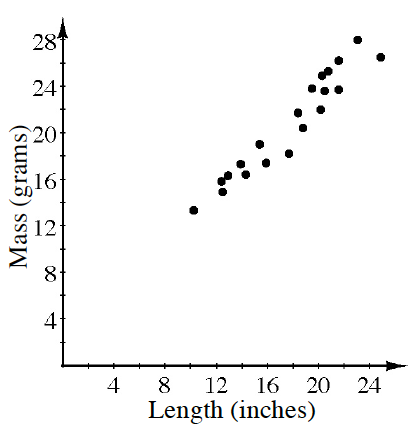Home > CC4 > Chapter 3 > Lesson 3.3.2 > Problem3-128

3-128.

The graph at right shows a comparison of the length of several gold chain necklaces (including the clasp) to the total mass. Homework Help ✎

1. Write an equation for the line of best fit. What are the units for slope and y‑intercept for your equation?

Eyeball a line of best fit. Then estimate the slope and $y$-intercept of your line.

$y = x + 2$

2. Based on your equation, what would you expect to be the mass of a $26$-inch chain?

• Substitute $26$ into your equation for $x$ and evaluate for $y$.